Numbers in Expanded & Word Form (3-Digit Numbers) - K-2nd Grade
1%
It was processed successfully!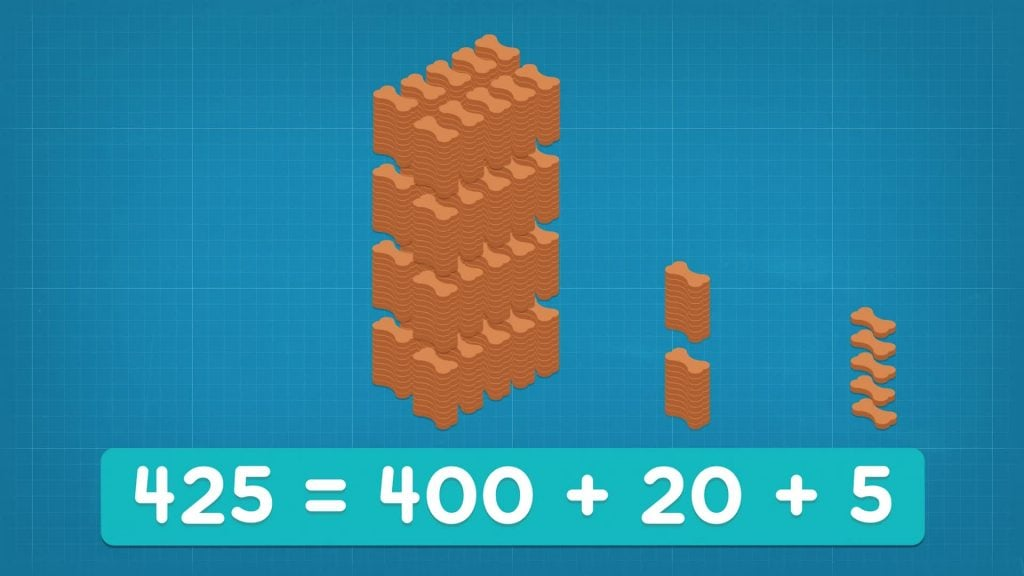WHAT IS WRITING NUMBERS IN DIFFERENT FORMS?

You can write numbers in different forms. Expanded form means writing the number so you show the value of each digit. Word form means writing the number with words instead of numerals.

To better understand numbers in expanded & word form…

WHAT IS WRITING NUMBERS IN DIFFERENT FORMS?. You can write numbers in different forms. Expanded form means writing the number so you show the value of each digit. Word form means writing the number with words instead of numerals. To better understand numbers in expanded & word form…

## LET’S BREAK IT DOWN!

### Review Place Value.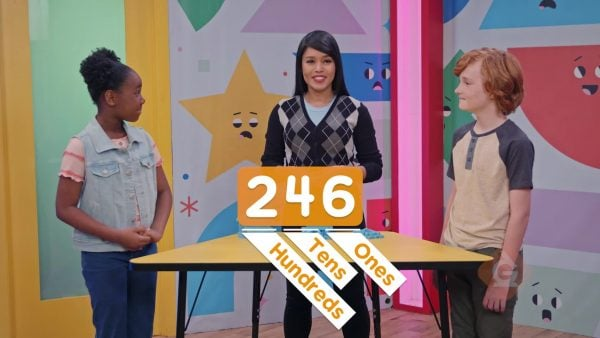Place value is the value of a digit based on its position in a number. 246 is 2 hundreds, 4 tens, and 6 ones.

Review Place Value. Place value is the value of a digit based on its position in a number. 246 is 2 hundreds, 4 tens, and 6 ones.

### Write Numbers in Expanded Form.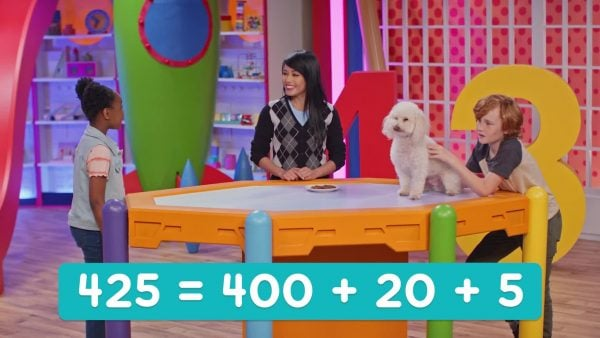Expanded form is a way to write a number that shows the value of each digit. In 425, the value of 4 is 400. The value of 2 is 20. The value of 5 is 5. In expanded form, 425 is 400 + 20 + 5.

Write Numbers in Expanded Form. Expanded form is a way to write a number that shows the value of each digit. In 425, the value of 4 is 400. The value of 2 is 20. The value of 5 is 5. In expanded form, 425 is 400 + 20 + 5.

### Write Numbers in Word Form.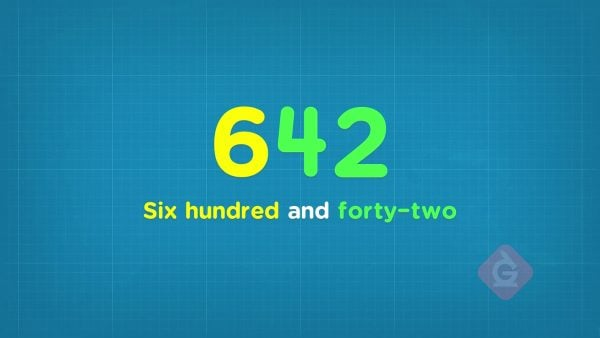You can also write numbers with words. Word form represents how you say a word when you read it out loud. In word form, 642 is “six hundred forty-two.” 214 is “two hundred fourteen.”

Write Numbers in Word Form. You can also write numbers with words. Word form represents how you say a word when you read it out loud. In word form, 642 is “six hundred forty-two.” 214 is “two hundred fourteen.”

### Write Expanded Form with Zeroes.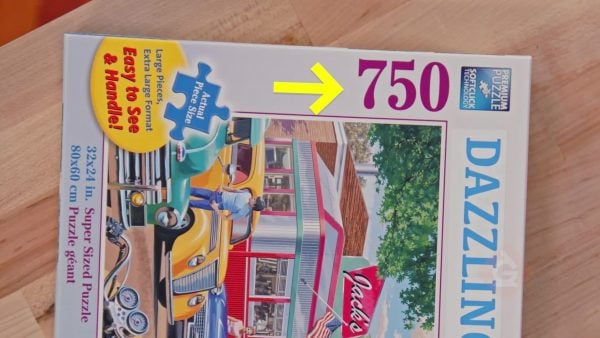Sometimes numbers have a 0 in them. 305 is 3 hundreds, 0 tens, and 5 ones. 305 in expanded form is 300 + 5. The expanded form doesn't show tens as there are 0 tens. 450 is 4 hundreds, 5 tens, and 0 ones, or 400 + 50.

Write Expanded Form with Zeroes. Sometimes numbers have a 0 in them. 305 is 3 hundreds, 0 tens, and 5 ones. 305 in expanded form is 300 + 5. The expanded form doesn't show tens as there are 0 tens. 450 is 4 hundreds, 5 tens, and 0 ones, or 400 + 50.There are no tens in 305. When reading 305, you say "three hundred five". There are no ones in 450. When reading 450, you say "four hundred fifty."

Reading Numbers with Zeroes. There are no tens in 305. When reading 305, you say "three hundred five". There are no ones in 450. When reading 450, you say "four hundred fifty."

## NUMBERS IN EXPANDED & WORD FORM (3-DIGIT NUMBERS) VOCABULARY

Numbers
Symbols used to show how many of something you have.
3-digit number
A 3-digit number is a number that has digits only in the hundred, tens, and ones places.
Place value
The value of a digit based on its position in a number.
Standard form of a number
The form of a number that uses only digits to show the value. For example, 631.
Expanded form
Another way to show the value of each digit in a number. To write a number in expanded form, write it as the sum of the values of its digits. For example, 631 = 600 + 30 + 1.
Word form
The name of a number. For example, the word form of 631 is six hundred thirty-one.

## NUMBERS IN EXPANDED & WORD FORM (3-DIGIT NUMBERS) DISCUSSION QUESTIONS

### How do you write a 3-digit number in expanded form?

Write the value of each digit. Then add them together. For example, in 123, the 1 represents a value of 100, the 2 represents a value of 20, and the 3 represents a value of 3. Add the values together to get the expanded form, 100 + 20 + 3.

### How do you write a 3-digit number in word form?

First, write the number of hundreds using words. Then, write the name of the number after the hundreds place. For example, 123 has a 1 in the hundreds place, so write “one hundred.” Then write the number after the hundreds place, “twenty-three.” In word form, 123 is one hundred twenty-three.

### What is 197 in expanded form and word form?

100 + 90 + 7; one hundred ninety-seven

### What is 310 in expanded form and word form?

300 + 10; three hundred ten

900 + 6; 906
X

## Success

We’ve sent you an email with instructions how to reset your password.
Ok